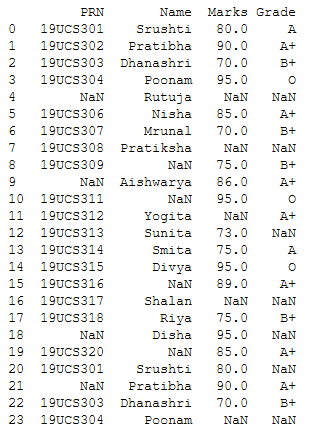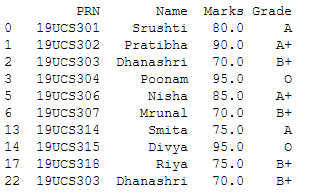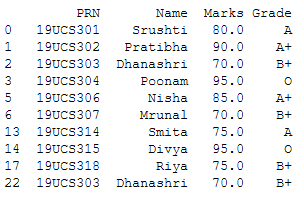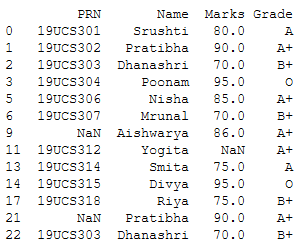# How to display notnull rows and columns in a Python dataframe?

• Last Updated : 03 Mar, 2021

In Python, not null rows and columns mean the rows and columns which have Nan values, especially in the Pandas library. To display not null rows and columns in a python data frame we are going to use different methods as dropna(), notnull(), loc[].

• dropna() : This function is used to remove rows and column which has missing values that are NaN values. dropna() function has axis parameter. If it set to 0 then it will remove all the rows which have NaN value and if it is set to 1 then it will remove all the columns which have NaN value. By default, the value of axis parameter is 0.
• notnull(): This function detects non-missing values and returns a mask of boolean values for each element in DataFrame that indicates whether an element is not an NA value.
• loc[]: This method filter rows and columns by labels or boolean array. In our example, this method filters rows by a boolean array which is returned by notnull() method.

Steps:

• Import pandas library
• Read the CSV file, or you can create your own data frame.
• Use one of the method like dropna(), notnull(), loc[] as described below.
• Display result

Below StudentData.csv file used in the program:Method 1: Using dropna() method

In this method, we are using the dropna() method which drops the null rows and displays the modified data frame.

## Python3

 `# Import library ` `import` `pandas as pd ` ` `  `# Reading csv file ` `df ``=` `pd.read_csv(``'StudentData.csv'``) ` ` `  `# using dropna() method ` `df ``=` `df.dropna() ` ` `  `# Printing result ` `print``(df) `

Output:Method 2: Using notnull() and dropna() method

In this method, we will first use notnull() method which is return a boolean object having True and False values. If there is NaN value it will return false otherwise true. And then give these boolean objects as input parameters to where function along with this function use drpna() to drop NaN rows.

## Python3

 `# Import library ` `import` `pandas as pd ` ` `  `# Reading csv file ` `df ``=` `pd.read_csv(``'StudentData.csv'``) ` ` `  `# using notnull() method ,it will return  ` `# boolean values ` `mask ``=` `df.notnull() ` ` `  `# using dropna() method to drop NaN  ` `# value rows ` `df ``=` `df.where(mask).dropna() ` ` `  `# Displaying result ` `print``(df) `

Output:Method 3: Using loc[] and notnull() method

In this method, we are using two concepts one is a method and the other is property. So first, we find a data frame with not null instances per specific column and then locate the instances over whole data to get the data frame.

## Python3

 `# Import library ` `import` `pandas as pd ` ` `  `# Reading csv file ` `df ``=` `pd.read_csv(``'StudentData.csv'``) ` ` `  `# Here filtering the rows according to  ` `# Grade column which has notnull value. ` `df ``=` `df.loc[df[``'Grade'``].notnull()] ` ` `  `# Displaying result ` `print``(df) `

Output:

As shown in the output image, only the rows having Grade != NaN is displayed.My Personal Notes arrow_drop_up
Recommended Articles
Page :